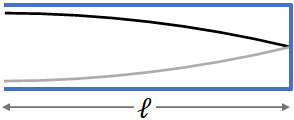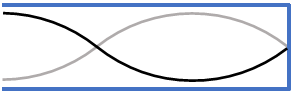﻿ Sound

# Sound

Sound is a vibration that propagates as a wave through material media such as air, liquids and solids. Sound waves can be produced by vibrating strings, vibrating membranes, vibrating metals, and vibrating air columns.

### Characteristics of Sound

Sound can travel through any kind of matter, but not through a vacuum. In gases and liquids sound transmits as longitudinal waves. Speed of sound is different in different materials: it is slowest in gases, faster in liquids, and fastest in solids. When sound waves propagate it makes particles in the medium oscillate but it does not carry with them.

#### Speed of sound in air

In air, speed of sound varies with temperature. Sound speed, $v$ increases with air temperature as

$v=331+0.60\,T$

where $T$ is the temperature in $^\circ C$ and $v$ is in $m/s$.

#### Intensity and loudness

Intensity of a sound wave is the energy it transports per unit time across unit area. Since power is energy per unit time, intensity is also the power per unit area. Loudness of sound is related to the intensity, higher the intensity, louder the sound. Human ear can detect sounds with an intensity as low as $10^{-12}\:W/m^2$. This intensity is called the threshold intensity of hearing.

#### Audible range

Pitch is the frequency of sound. Human ears can hear only the sound with frequencies in the range $20 Hz$ to $20kHz$. This is called the audible range.

#### Perceived loudness and decibels

Although the loudness depends on the intensity of the sound, perceived loudness, is not linearly proportional to the intensity of the sound, but it is closely related to the logarithm of the intensity. So a logarithmic scale is commonly used for sound level with unit of decibels (dB). Sound level in decibels $(dB)$ is defined as

$\,\beta (dB)=10\log\left( \dfrac{I}{I_0}\right)$

where $I$ is the intensity of the sound and $I_0$ is the threshold intensity of hearing. i.e., $I_0=10^{-12}\:W/m^2$

#### Sound intensity versus distance

As with any other waves, intensity of sound diminishes with distance. If you move away from the source, the sound intensity decreases with distance as an inverse square law,

$I=\dfrac{P}{4\pi r^2}$

where $P$ is the power output of the sound source and $r$ is the distance from the source.

#### Sources of Sound

Stringed instruments such as guitars produce sound waves by vibrating plucked strings and wind instruments such as flutes produce sound by blowing and subsequent vibration of the air column withing the instruments.

#### Vibrating string resonance

A plucked string in a musical instrument can vibrate only at certain frequencies, called the resonant frequencies of the string. These frequencies are the frequencies of the standing waves on the string. So, a stringed instrument can produce sound of frequencies at the resonant frequencies of the string.

Resonant wavelengths on a stretched string are

$\lambda_n=\dfrac{2l}{n}$ where $n=1,2,3 ...$

and

Resonant frequencies are

$f_n=n \dfrac{v}{2l}$ where $n=1,2,3, ...$

The frequency corresponds to $n=1$, i.e., $f_1=\dfrac{v}{2l}$ is called the fundamental frequency. All other frequencies (except the fundamental) are called overtones. All resonant frequencies corresponds to $n=1,2,3, ...$, i.e., $f_1,f_2,f_3, ...$ are called are called harmonics.

#### Wind instruments

A wind instrument create sound through standing waves in a column of air. A wind instrument can be a closed tube, a tube closed at one end or an open tube, a tube open at both ends.

#### Closed tube resonance

In a tube closed at one end, the air molecules cannot vibrate at the closed end. This restriction limits the possible resonant frequencies of the tube. The first three possible standing waves in a closed tube are as follows:$l=\lambda_1/4$ There is a quarter of the wave over the length of the tube, $l$ therefore,

$l=\lambda_1/4$

or

$\lambda_1= 4l$$l=3\,\lambda_2/4$ There is a 3/4 th of the wave in the tube therefore,

$l=3\,\lambda_2/4$

or

$\lambda_2= 4l/3$

Here, there are 1 and a quarter wave, therefore,

$l=5\,\lambda_3/4$

or

$\lambda_3= 4l/5$

Like these there are infinite number of possible resonant wavelengths. If we combine those equations, we will end up with an equation:

$\lambda_n=\dfrac{4l}{n}$, where $n=1,3, 5,7,...$

By using $v=f\lambda$, we can write the resonant frequencies of the closed tube:

$f_n=n\dfrac{v}{4l}$, where $n=1,3,5,7, ...$

The frequency corresponds to $n=1$ is the fundamental frequency. All resonant frequencies are called harmonics. Frequencies other than the fundamental are called overtones.

#### Open tube resonance

In a tube open at both ends, when blown, the air molecules always vibrate with higher amplitudes at the end of the tubes. Due to this, the resonant frequencies of the tube are restricted to certain frequencies. The first three possible standing waves in a open tube are given below. There is a half wave over the length of the tube, so we have

$l=\lambda_1/2$

or

$\lambda_1= 2l$

Here, there is a full wave over the tube's length, so we have

$l=\lambda_2$

or

$\lambda_2= l$

In the above picture, there are 1 and a half wave, and therefore

$l=3\,\lambda_3/2$

or

$\lambda_3= 2\,l/3$

Combining the above equations, we can write,

$\lambda_n=\dfrac{2l}{n}$, where $n=1,3, 4,...$

These are the resonant wavelengths of an open tube. From this equation, we can write the resonant frequencies,

$f_n=n\dfrac{v}{2l}$, where $n=1,2,3,...$

### Doppler Effect

When a source of sound is moving toward a stationary observer, the pitch of sound the observer hears is higher than that when the source is at rest. And, when the source is moving away from the observer, the pitch is lower. This phenomenon is called Doppler effect. You can experience Doppler effect with the siren of a fire truck, a police car or an ambulance. When a fire truck approaches you, you hear the pitch of its siren increases and as it passes by and move away from you, you hear the decrease of pitch.

Doppler effect depends on the velocity of the source, the velocity of the observer (if the observer is moving) and the direction of their motion.

Consider a fire truck that is in rest blasts its siren. It sends out sound waves in all directions. The crest of the sound waves are shown as green lines in the figure. Since the truck is in rest, if you look at the front or the back or any where around the truck, a new wave crest arrives in every $T$ seconds at a given location in space, where $T$ is the period of the sound wave. The successive crests are separated by a distance equal to the wavelength $\lambda$ of the sound.

So, when the truck is in rest, the sound waves emitted by the siren of the truck is same in all directions. But the things change when the truck starts moving, as you see in the picture below. Let us consider a crest that comes out from the source, so the next crest will be out after $T$ seconds. Now, if you look in front of the truck, before the next crest leave the source, the truck moves a distance closer to the previous crest, by $d$, where $d = v_s T$, and $v_s$, the velocity of the source (the truck). The result is, the crests are much closer in front of the truck. So the wavelength of the wave is shorter in front of the truck. Smaller wavelength means, higher frequency and the person in front of the truck hears a high pitch sound. But for a person behind the truck, wavelength is longer, and so the frequency or the pitch is lower.

Now, let us find the frequency of the sound heard by a stationary observer.

#### source is moving toward a stationary observer

When the source is moving toward a stationary observer, as we saw, the wavelength shortens by $d$, the distance the source travels during the time $T$. If we take $\lambda'$ is the wavelength of the sound in front of the moving source, we have

$\lambda'=\lambda-d$

or

$\lambda'=\lambda-v_sT$

Now, we will change the wavelengths into frequencies by using $v=f\lambda$

i.e., $\lambda = v/f$ and $\lambda' = v/f'$, we get

$\dfrac{v}{f'} =\dfrac{v}{f}-\dfrac{v_s}{f}$

Solving for $f'$,

$f'=\dfrac{f}{1-\dfrac{v_s}{v}}$

This is the frequency of sound observed by a stationary observer when the source of sound move toward the observer.

If the source is moving away from the stationary observer, then $v_s$ will be negative, and we will have

$f'=\dfrac{f}{1+\dfrac{v_s}{v}}$

This is the frequency of the sound observed by a stationary observer when the source of sound move away from the observer.

#### Stationary source and observer move towards the source

When the source is stationary, the wavelength of the sound from the source does not change. But since the wave is moving toward the observer and the observer is moving opposite to the wave, the speed of the wave relative to the observer is now, $v'=v+v_{obs}$, where $v_{obs}$ is the velocity of the observer. So, the frequency of the sound as observed by the observer is

$f'=v'/\lambda$

$f'=(v+v_{obs})/\lambda$

or

$f'=f(v+v_{obs})/v$

or

$f'=f\left(1+\dfrac{v_{obs}}{v}\right)$

If the observer is moving away from the stationary source, the velocity direction changes, therefore, we need to add a minus sign in the above equation:

$f'=f\left(1-\dfrac{v_{obs}}{v}\right)$

If we combine all the four equations that we derived above, we can write a single equation for the observed frequency

$f'=f\left(\dfrac{v\pm v_{obs}}{v\mp v_s}\right)$

This equation is valid, whether the source is moving, or the observer is moving or both of them are moving.

In the above equation, when source (and/or) observer move toward each other, you should have a $+$ sign in the numerator and a $−$ sign in the denominator. But when source (and/or) observer move away from each other, switch the sign, i.e., $-$ in the numerator and a $+$ sign in the denominator.